# Solution assignment 09 Quadratic functions and graphs

### Assignment 9

Calculate the equation of the parabola going through the points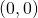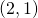and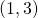.

### Solution

The general equation of a parabola is:We have to calculate the values of,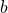and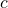based on the fact that the given points lie on the parabola.lies on the parabola, thus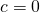.lies on the parabola, thus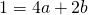lies on the parabola, thus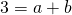So we have two solve two linear equations with two variablesand: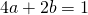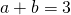Multiply the second equation by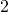and subtract the result from the first equation (in this way we eliminate):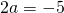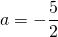If we substitute this value ofin the second equation we get for: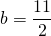0